# Triangles and their Congruence

Go back to  'Geometry'

## What is a triangle?

A triangle is a closed figure with 3 sides and 3 vertices.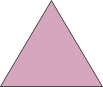## Classification of triangles by sides

### Equilateral triangle

If all the three sides of a triangle are equal, it is called an equilateral triangle.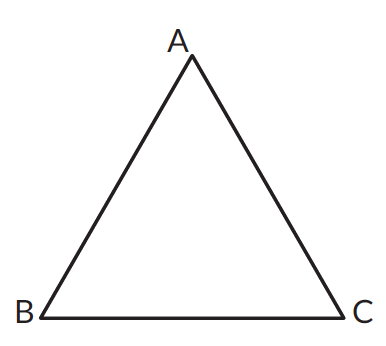All three sides are equal in length. $$AB = BC = AC$$

### Isosceles triangle

If any two sides of a triangle are equal, it is called an isosceles triangle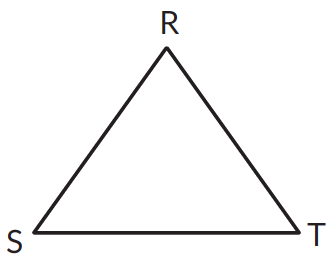Two sides of this triangle are equal. $$RS = RT$$

### Scalene triangle

If a triangle has three unequal sides, it is called a scalene triangle.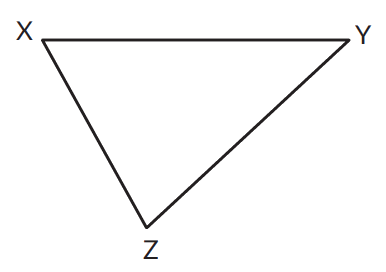In this triangle all three sides are of unequal lengths. $$XZ ≠ XZ ≠ YZ$$

## Classification of triangles by angles

### Right angled triangle

If any angle of a triangle is $$90^\circ$$, the triangle is called a right-angled triangle or a right triangle.$$\angle ABC = 90^\circ$$. Therefore $$\triangle ABC$$ is a right-angled triangle.

### Obtuse angled triangle

If any angle of a triangle is obtuse, then the triangle is called an obtuse-angled triangle or an obtuse triangle.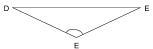$$\angle DEF$$ is an obtuse angle. Therefore $$\triangle DEF$$ is an obtuse-angled triangle.

### Acute angled triangle

If every angle of a triangle is acute, then the triangle is called an acute-angled triangle or an acute triangle.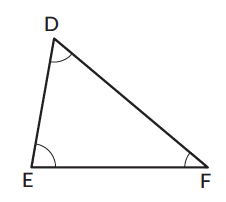All the angles, $$\angle DEF$$, $$\angle EFD$$, and $$\angle EDF$$ are all acute angles. Therefore, the triangle is an acute-angled triangle.

## Procedure - Angle sum property of a triangle

The sum of the angles of a triangle is $$180^\circ$$. This property is called the angle sum property of a triangle. All the interior angles sum up to $$180^\circ$$.

Let’s try and understand this with the help of an example.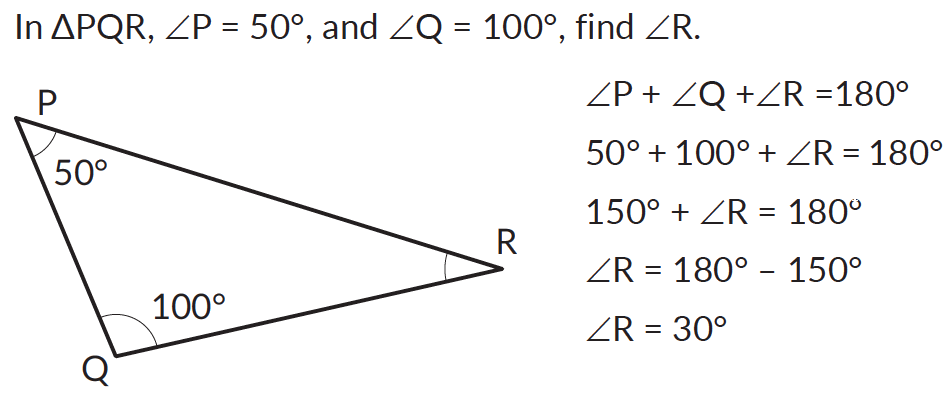## Triangle inequality property

In every triangle, the sum of any two sides is greater than the third side. This is the triangle inequality property.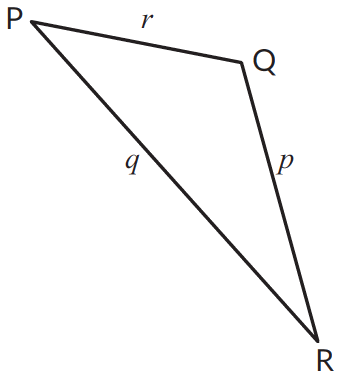In the given figure,
$$p + q \gt r$$
$$q + r \gt p$$
$$r + p \gt q$$

## Procedure - Pythagoras theorem

Consider a right-angled triangle with sides $$a$$, $$b$$, and $$c$$.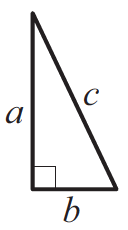Here are four copies of the triangle oriented differently.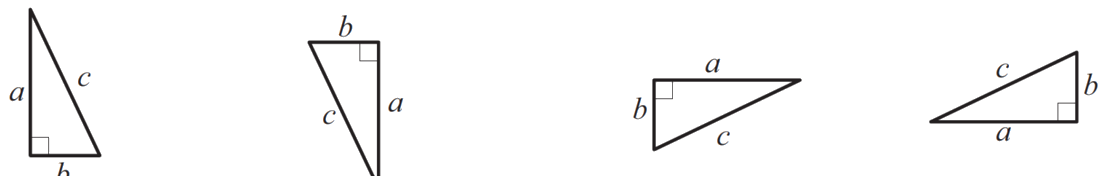Putting all these together to form a square. This is a square with side $$(a + b)$$.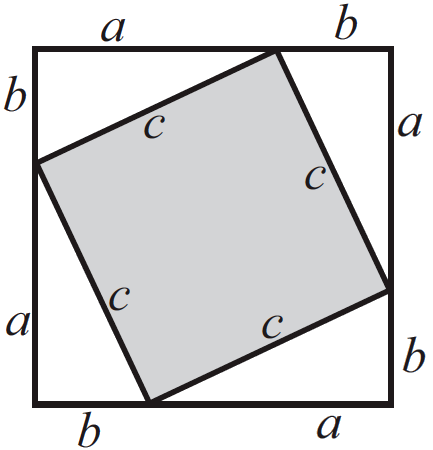The shaded region is also a square. Why?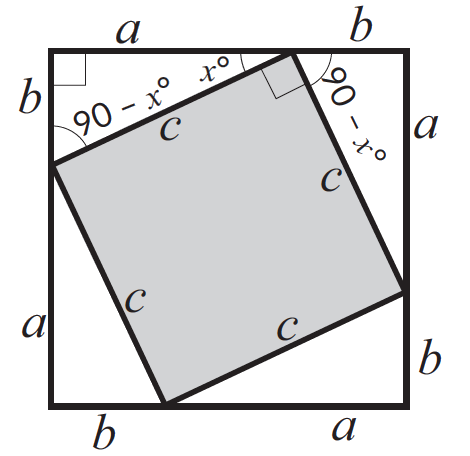In the above figure, there are four triangles. Each triangle's area is \begin{align}{1\over 2} \times {b \times h}\end{align}

$$\therefore$$The total area of the four triangles $$= (2ab)$$

The area of the inside (shaded) square $$= c^2$$

The area of the larger square of side $$(a + b) = (a + b)^2$$

The area of the larger square $$=$$ the total area of the four triangles $$+$$ the area of the inside square

\begin{align}(a + b)^2 & = (2ab) + c^2\\a^2 + b^2 & = c^2\end{align}

In a right-angled triangle, the square of the hypotenuse (the longest side) equals the sum of the squares of the other two sides.

In $$ABC$$,

\begin{align}(AB)^2 + (BC)^2 &= (AC)^2\\\AB)^2 &= (AC)^2 - (BC)^2 \qquad \qquad \quad \;\, (BC)^2 = (AC)^2 - (AB)^2\\\\AB &= \sqrt{(AC)^2-(BC)^2} \qquad \qquad BC = \sqrt{(AC)^2-(AB)^2}\end{align} ## Concept of congruence When two figures can be perfectly overlapped, they are said to be congruent to each other.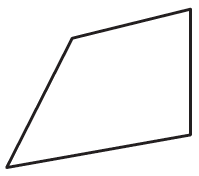Two line segments are congruent when their lengths are equal.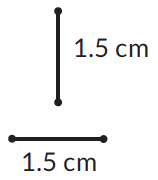Two angles are congruent when they are equal.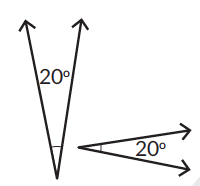## Procedure - Congruence of triangles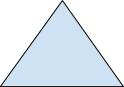By definition, all the corresponding sides and angles of two congruent triangles are equal. This means six measures are equal. However, we can prove congruence using fewer than six measures! ### SAS Criterion Consider the two figures,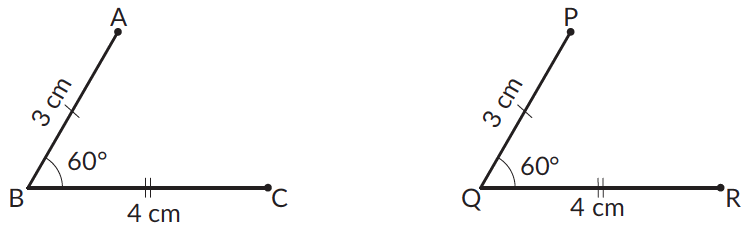\(\begin{align} & AB = PQ = 3\text{ cm,} \\ & BC = QR = 4\text{ cm,}\\ & \angle B = \angle Q = 60^\circ \end{align}

Let us now close these shapes to compare them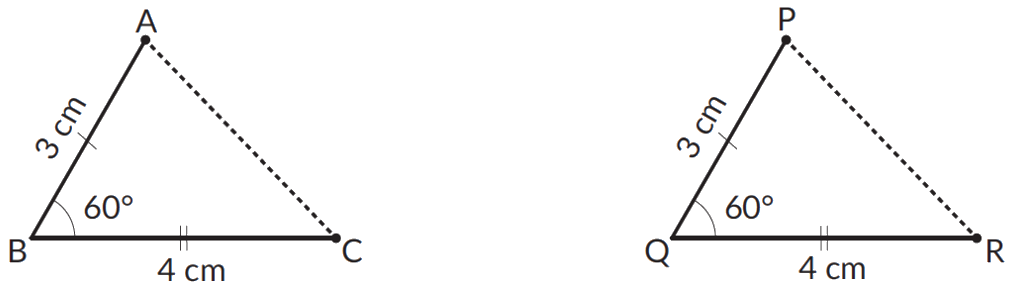The two triangles overlap and \begin{align} \triangle ABC ≅ \triangle PQR \end{align}

If two sides and the included angle of one triangle are equal to two corresponding sides and the included angle of another triangle, the triangles are congruent by the Side-Angle-Side or the SAS criterion.

### SSS Criterion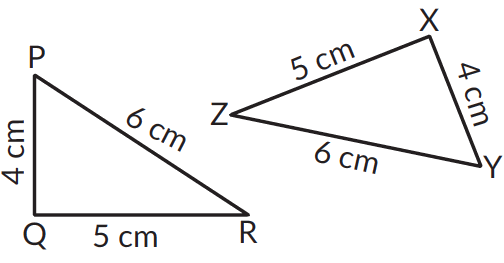### RHS Criterion

Consider two right-angled triangles $$\triangle ABC$$ and $$PQR$$.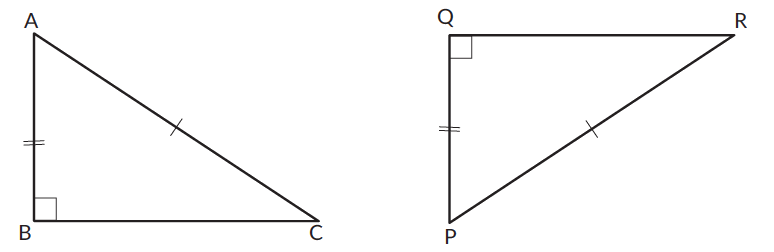Here $$AC = PR$$, $$AB = QP$$ and $$\angle B = \angle Q = 90^\circ$$

Using the Pythagoras theorem

Find $$BC$$ in $$\triangle ABC$$

\begin{align} BC = \sqrt{(AC^2)-(AB^2)} \end{align}

Find $$QR$$ in $$\triangle PQR$$

\begin{align} QR = \sqrt{(PR^2)-(PQ^2)} \end{align}

We know, $$QP = AB$$ and $$PR = AC$$.

\begin{align} QR = \sqrt{(AC^2)-(AB^2)} \end{align}

$$BC = QR$$. The three corresponding sides of both the triangles equal to each other. \begin{align} \triangle ABC ≅ \triangle PQR \end{align}.

If the hypotenuse and a side of a right-angled triangle are equal to the hypotenuse and corresponding side of another right-angled triangle, the triangles are congruent by the Right-angle-Hypotenuse-Side or the RHS criterion.

### ASA Criterion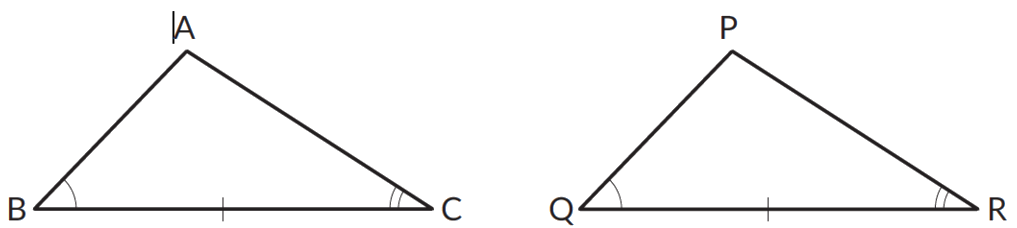$$\angle ABC = \angle PQR$$, $$\angle BCA = \angle QRP$$ and $$BC = QR$$

If we show that $$AB = PQ$$, then the triangles are congruent by ASA congruence criterion.

Let us assume that $$AB ≠ PQ$$. If $$AB \gt PQ$$, then let $$BD = PQ$$, where $$D$$ lies on $$AB$$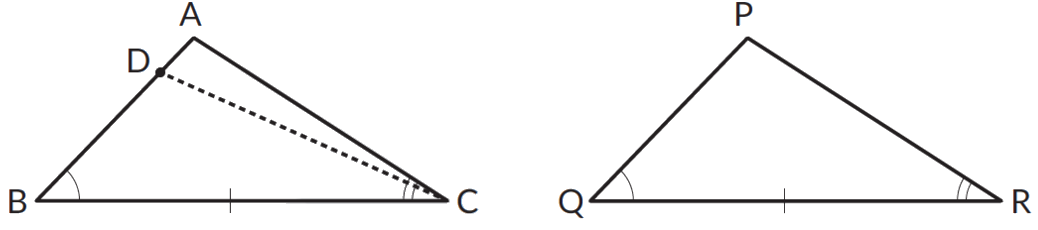\begin{align} & \triangle BCD ≅ \triangle QRP \\ & \angle BCD = \angle QRP \end{align}

Also, $$\angle BCA = \angle QRP$$

$$\angle BCA = \angle BCD$$, which means that the points $$D$$ and $$A$$ must coincide

$$AB = DB = PQ$$

Now, using the SAS criterion, we can say that $$\triangle ABC ≅ \triangle ABC$$

If two angles and the included side of a triangle are equal to two corresponding angles and a side of another triangle, the triangles are congruent by the Angle-Side-Angle or the ASA criterion.

## Tips and Tricks

1. Tip: Children are used to seeing triangles in a single orientation, that the vertex at the top and 2 vertices at the bottom. It would help showing the child to look at triangles in different orientations. Draw triangles in as many orientations as possible.

### Common mistakes or misconceptions

Misconception 1: All triangles have a vertex on the top and 2 vertices at the bottom.

Triangles can be in any orientation. For example, the shapes given below are all triangles. They are closed figures made of 3 line segments.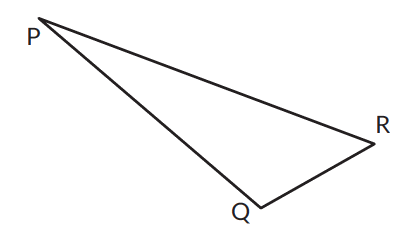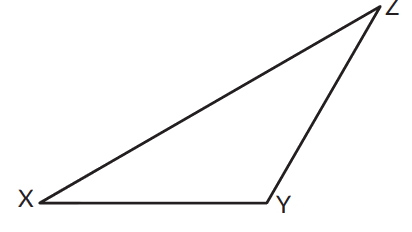Misconception 2: Equilateral triangle is not an isosceles triangle.

All sides in an equilateral triangle are equal. Hence every equilateral triangle is an isosceles triangle. But not every isosceles triangle is an equilateral triangle.

1. What is the measure of $$\angle N$$?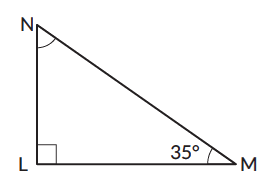1. Can angles of measure $$40^\circ$$, $$30^\circ$$, and $$70^\circ$$ be the three angles of a triangle?
Yes                No
2. The three angles of a triangle measure $$7^\circ$$, $$164^\circ$$, and $$9^\circ$$. What kind of triangle is it?
3. All three angles of a triangle are equal. What is the measure of each angle?
4. At most, how many obtuse angles can a triangle have?